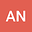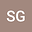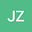Mathematics of circulation in arbitrary fluid property spaces
•••• +1## Abstract

Projecting fluid systems onto coordinates defined by fluid properties (e.g., pressure, temperature, tracer concentration) can reveal deep insights, for example into the thermodynamics and energetics of the ocean and atmosphere. We present a mathematical formalism for fluid flow in such coordinates. We formulate mass conservation, streamfunction, tracer conservation, and tracer angular momentum within fluid property space (q-space) defined by an arbitrary number of continuous fluid properties. Points in geometric position space (x-space) do not generally correspond in a 1-to-1 manner to points in q-space. We therefore formulate q-space as a differentiable manifold, which allows differential and integral calculus but lacks a metric, thus requiring exterior algebra and exterior calculus. The Jacobian, as the ratio of volumes in x-space and q-space, is central to our theory. When x-space is not 1-to-1 with q-space, we define a generalized Jacobian either by patching x-space regions that are 1-to-1 with q-space, or by integrating a Dirac delta to select all x-space points corresponding to a given q value. The latter method discretises to a binning algorithm, providing a practical framework for analysis of fluid motion in arbitrary coordinates. Considering q-space defined by tracers, we show that tracer diffusion and tracer sources drive motion in q-space, analogously to how internal stresses and external forces drive motion in x-space. Just as the classical angular momentum of a body is unaffected by internal stresses, the globally integrated tracer angular momentum is unaffected by tracer diffusion — unless different tracers are diffused differently, as in double diffusion.